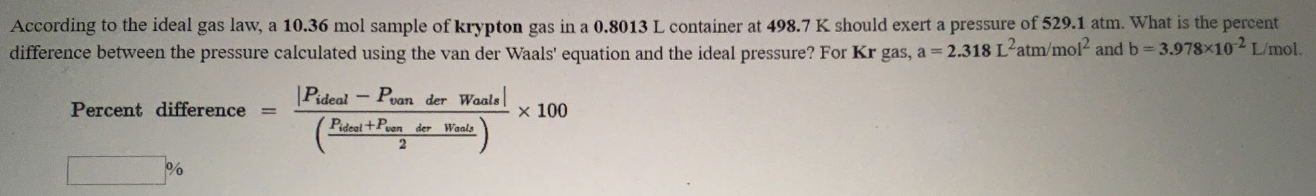# According to the ideal gas law, a 10.36 mol sample of krypton gas is 0.8013 L container at 498.7 K should exert a pressure of 529.1 atm. What is the percent difference between the pressure calculated using the van der Waal's equation and the ideal pressure? For Kr gas, a = 2.138 L^2atm/mol^2 and b = 3.978x10^-2 L/mol.